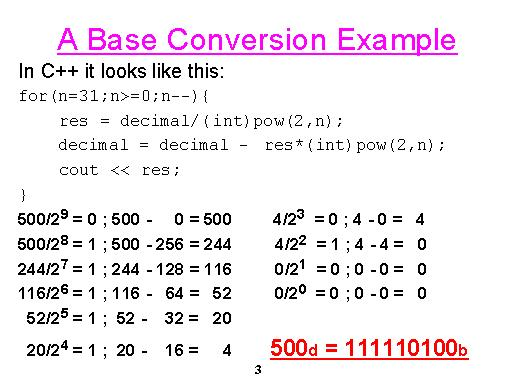# Assignment #1

Write a program that receives an integer and prints it in binary.
There are 2 ways to perform this:
1. Using a mask and the shift operator (<<) print each bit of the 32-bit integer.
2. Convert the number to binary using the following algorithm:Use both ways in your program. The program should work for both negative and positive numbers.
(Hint: There is no need to perform two's-complement conversion.)